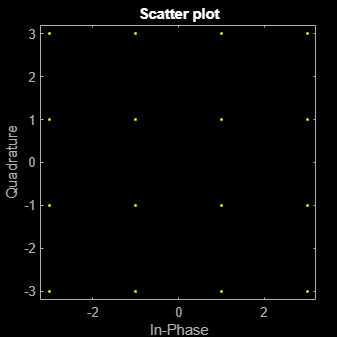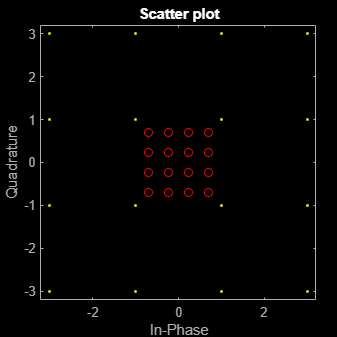Documentation

This is machine translation

Mouseover text to see original. Click the button below to return to the English version of the page.

modnorm

Scaling factor for normalizing modulation output

Syntax

``normfactor = modnorm(refconst,type,power)``

Description

example

````normfactor = modnorm(refconst,type,power)` returns a scale factor for normalizing a PAM or QAM modulator output using the specified reference constellation, normalization type, and output power.```

Examples

collapse all

Generate a 16-QAM reference constellation.

`refconst = qammod(0:15,16);`

Generate random symbols and apply 16-QAM modulation.

```x = randi([0 15],1000,1); y = qammod(x,16);```

Plot the constellation.

`h = scatterplot(y);`Compute the normalization factor so that the output signal has a peak power of 1 W.

```nf = modnorm(refconst,'peakpow',1); z = nf*y;```

Confirm that no element of the normalized signal has a power greater than 1 W.

`max(z.*conj(z))`
```ans = 1.0000 ```

Plot the scatter plot of the normalized constellation.

```hold on scatterplot(z,1,0,'r+',h) hold off```Input Arguments

collapse all

Reference constellation, specified as a vector of complex elements that comprise the reference constellation points.

Example: `qammod(0:15,16)`

Data Types: `double` | `single`
Complex Number Support: Yes

Normalization type, specified as either `'avpow'` or `'peakpow'`.

• If `type` is `'avpow'`, the normalization factor is calculated based on average power.

• If `type` is `'peakpow'`, the normalization factor is calculated based on peak power.

Data Types: `char`

Target power, specified as a real scalar. The target power is the intended power of the modulated signal multiplied by `normfactor`.

Data Types: `double` | `single`

Output Arguments

collapse all

Normalization factor, returned as a real scalar. When a modulated signal is multiplied by the normalization factor, its average or peak power matches the target power. The function assumes that the signal you want to normalize has a minimum distance of 2.

Data Types: `double` | `single`